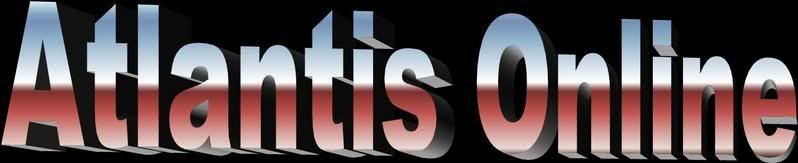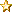August 20, 2019, 06:55:50 am
 Welcome, Guest. Please login or register. 1 Hour 1 Day Forever Login with username, password and session length
 News: Giant crater may lie under Antarctic icehttp://space.newscientist.com/article/dn9268Home Help Arcade Gallery Links Staff List Calendar Login Register

# Turing Machines and Universes

 Pages:    Go DownAuthor Topic: Turing Machines and Universes  (Read 25 times)
Raven
Hero MemberPosts: 573« on: August 04, 2007, 01:11:22 am »

Turing Machines and Universes

By: Dr. Sam Vaknin

In 1936 an American (Alonzo Church) and a Briton (Alan M. Turing) published independently (as is often the coincidence in science) the basics of a new branch in Mathematics (and logic): computability or recursive functions (later to be developed into Automata Theory).

The authors confined themselves to dealing with computations which involved "effective" or "mechanical" methods for finding results (which could also be expressed as solutions (values) to formulae). These methods were so called because they could, in principle, be performed by simple machines (or human-computers or human-calculators, to use Turing's unfortunate phrases). The emphasis was on finiteness: a finite number of instructions, a finite number of symbols in each instruction, a finite number of steps to the result. This is why these methods were usable by humans without the aid of an apparatus (with the exception of pencil and paper as memory aids). Moreover: no insight or ingenuity were allowed to "interfere" or to be part of the solution seeking process.

What Church and Turing did was to construct a set of all the functions whose values could be obtained by applying effective or mechanical calculation methods. Turing went further down Church's road and designed the "Turing Machine"  a machine which can calculate the values of all the functions whose values can be found using effective or mechanical methods. Thus, the program running the TM (=Turing Machine in the rest of this text) was really an effective or mechanical method. For the initiated readers: Church solved the decision-problem for propositional calculus and Turing proved that there is no solution to the decision problem relating to the predicate calculus. Put more simply, it is possible to "prove" the truth value (or the theorem status) of an expression in the propositional calculus  but not in the predicate calculus. Later it was shown that many functions (even in number theory itself) were not recursive, meaning that they could not be solved by a Turing Machine.

No one succeeded to prove that a function must be recursive in order to be effectively calculable. This is (as Post noted) a "working hypothesis" supported by overwhelming evidence. We don't know of any effectively calculable function which is not recursive, by designing new TMs from existing ones we can obtain new effectively calculable functions from existing ones and TM computability stars in every attempt to understand effective calculability (or these attempts are reducible or equivalent to TM computable functions).

The Turing Machine itself, though abstract, has many "real world" features. It is a blueprint for a computing device with one "ideal" exception: its unbounded memory (the tape is infinite). Despite its hardware appearance (a read/write head which scans a two-dimensional tape inscribed with ones and zeroes, etc.)  it is really a software application, in today's terminology. It carries out instructions, reads and writes, counts and so on. It is an automaton designed to implement an effective or mechanical method of solving functions (determining the truth value of propositions). If the transition from input to output is deterministic we have a classical automaton  if it is determined by a table of probabilities  we have a probabilistic automaton.

With time and hype, the limitations of TMs were forgotten. No one can say that the Mind is a TM because no one can prove that it is engaged in solving only recursive functions. We can say that TMs can do whatever digital computers are doing  but not that digital computers are TMs by definition. Maybe they are  maybe they are not. We do not know enough about them and about their future.

Moreover, the demand that recursive functions be computable by an UNAIDED human seems to restrict possible equivalents. Inasmuch as computers emulate human computation (Turing did believe so when he helped construct the ACE, at the time the fastest computer in the world)  they are TMs. Functions whose values are calculated by AIDED humans with the contribution of a computer are still recursive. It is when humans are aided by other kinds of instruments that we have a problem. If we use measuring devices to determine the values of a function it does not seem to conform to the definition of a recursive function. So, we can generalize and say that functions whose values are calculated by an AIDED human could be recursive, depending on the apparatus used and on the lack of ingenuity or insight (the latter being, anyhow, a weak, non-rigorous requirement which cannot be formalized).

Quantum mechanics is the branch of physics which describes the microcosm. It is governed by the Schrodinger Equation (SE). This SE is an amalgamation of smaller equations, each with its own space coordinates as variables, each describing a separate physical system. The SE has numerous possible solutions, each pertaining to a possible state of the atom in question. These solutions are in the form of wavefunctions (which depend, again, on the coordinates of the systems and on their associated energies). The wavefunction describes the probability of a particle (originally, the electron) to be inside a small volume of space defined by the aforementioned coordinates. This probability is proportional to the square of the wavefunction. This is a way of saying: "we cannot really predict what will exactly happen to every single particle. However, we can foresee (with a great measure of accuracy) what will happen if to a large population of particles (where will they be found, for instance)."

This is where the first of two major difficulties arose:

To determine what will happen in a specific experiment involving a specific particle and experimental setting  an observation must be made. This means that, in the absence of an observing and measuring human, flanked by all the necessary measurement instrumentation  the outcome of the wavefunction cannot be settled. It just continues to evolve in time, describing a dizzyingly growing repertoire of options. Only a measurement (=the involvement of a human or, at least, a measuring device which can be read by a human) reduces the wavefunction to a single solution, collapses it.

A wavefunction is a function. Its REAL result (the selection in reality of one of its values) is determined by a human, equipped with an apparatus. Is it recursive (TM computable and compatible)? In a way, it is. Its values can be effectively and mechanically computed. The value selected by measurement (thus terminating the propagation of the function and its evolution in time by zeroing its the other terms, bar the one selected) is one of the values which can be determined by an effective-mechanical method. So, how should we treat the measurement? No interpretation of quantum mechanics gives us a satisfactory answer. It seems that a probabilistic automaton which will deal with semi recursive functions will tackle the wavefunction without any discernible difficulties  but a new element must be introduced to account for the measurement and the resulting collapse. Perhaps a "boundary" or a "catastrophic" automaton will do the trick.

The view that the quantum process is computable seems to be further supported by the mathematical techniques which were developed to deal with the application of the Schrodinger equation to a multi-electron system (atoms more complex than hydrogen and helium). The Hartree-Fok method assumes that electrons move independent of each other and of the nucleus. They are allowed to interact only through the average electrical field (which is the charge of the nucleus and the charge distribution of the other electrons). Each electron has its own wavefunction (known as: "orbital")  which is a rendition of the Pauli Exclusion Principle.

The problem starts with the fact that the electric field is unknown. It depends on the charge distribution of the electrons which, in turn, can be learnt from the wavefunctions. But the solutions of the wavefunctions require a proper knowledge of the field itself!

Thus, the SE is solved by successive approximations. First, a field is guessed, the wavefunctions are calculated, the charge distribution is derived and fed into the same equation in an ITERATIVE process to yield a better approximation of the field. This process is repeated until the final charge and the electrical field distribution agree with the input to the SE.

Recursion and iteration are close cousins. The Hartree-Fok method demonstrates the recursive nature of the functions involved. We can say the SE is a partial differential equation which is solvable (asymptotically) by iterations which can be run on a computer. Whatever computers can do  TMs can do. Therefore, the Hartree-Fok method is effective and mechanical. There is no reason, in principle, why a Quantum Turing Machine could not be constructed to solve SEs or the resulting wavefunctions. Its special nature will set it apart from a classical TM: it will be a probabilistic automaton with catastrophic behaviour or very strong boundary conditions (akin, perhaps, to the mathematics of phase transitions).

Classical TMs (CTMs, Turing called them Logical Computing Machines) are macroscopic, Quantum TMs (QTMs) will be microscopic. Perhaps, while CTMs will deal exclusively with recursive functions (effective or mechanical methods of calculation)  QTMs could deal with half-effective, semi-recursive, probabilistic, catastrophic and other methods of calculations (other types of functions).

The third level is the Universe itself, where all the functions have their values. From the point of view of the Universe (the equivalent of an infinite TM), all the functions are recursive, for all of them there are effective-mechanical methods of solution. The Universe is the domain or set of all the values of all the functions and its very existence guarantees that there are effective and mechanical methods to solve them all. No decision problem can exist on this scale (or all decision problems are positively solved). The Universe is made up only of proven, provable propositions and of theorems. This is a reminder of our finiteness and to say otherwise would, surely, be intellectual vanity.

--------------------------------------------------------------------------------
http://samvak.tripod.com/turing.html
 Report SpamLogged

 Pages:    Go Up
Jump to:

Powered by EzPortal